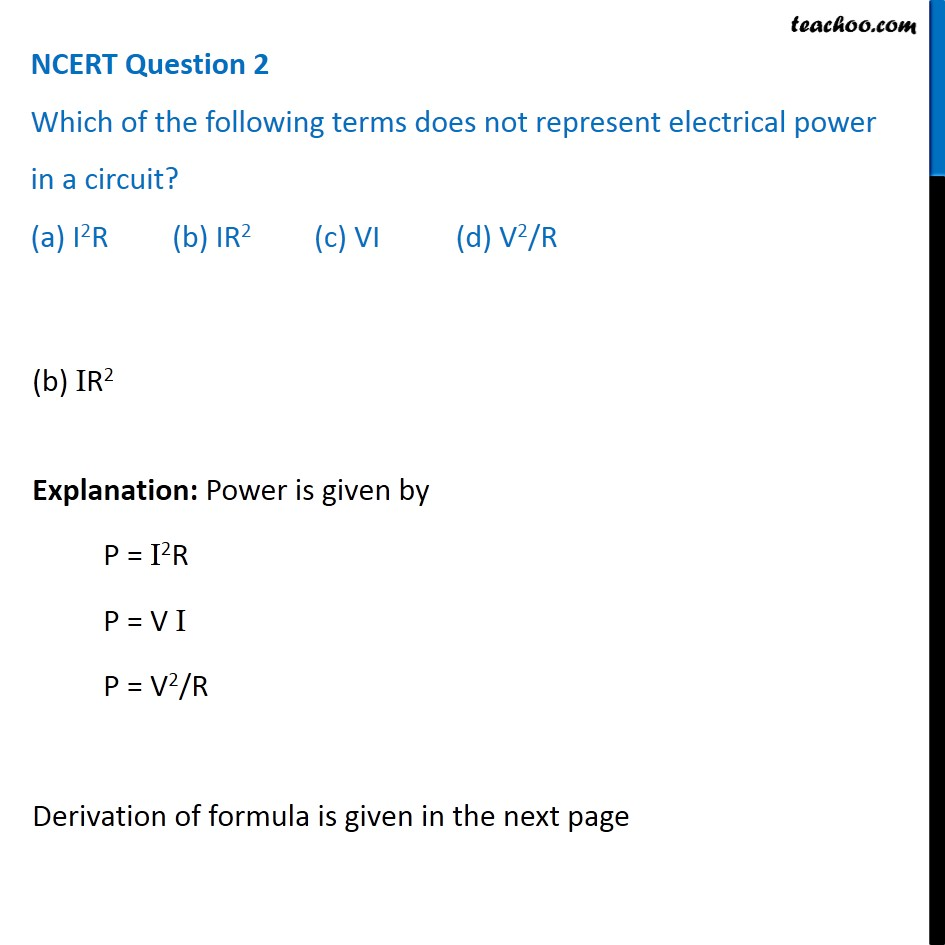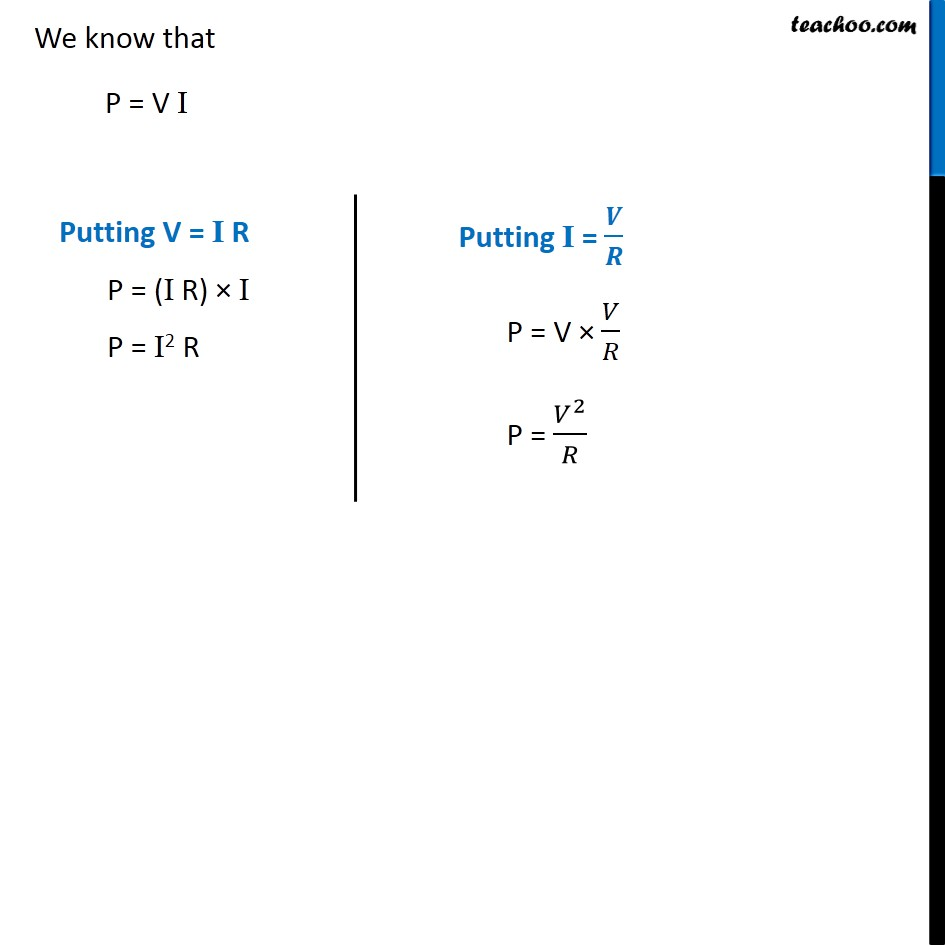NCERT Questions

Class 10
Chapter 12 Class 10 - Electricity

## Which of the following terms does not represent electrical power in a circuit? (a) I 2 R (b) IR 2 (c) VI (d) V 2 /RLearn in your speed, with individual attention - Teachoo Maths 1-on-1 Class

### Transcript

NCERT Question 2 Which of the following terms does not represent electrical power in a circuit? (a) I2R (b) IR2 (c) VI (d) V2/R (b) IR2 Explanation: Power is given by P = I2R P = V I P = V2/R Derivation of formula is given in the next page We know that P = V I Putting V = I R P = (I R) × I P = I2 R Putting I = 𝑽/𝑹 P = V × 𝑉/𝑅 P = 𝑉^2/𝑅